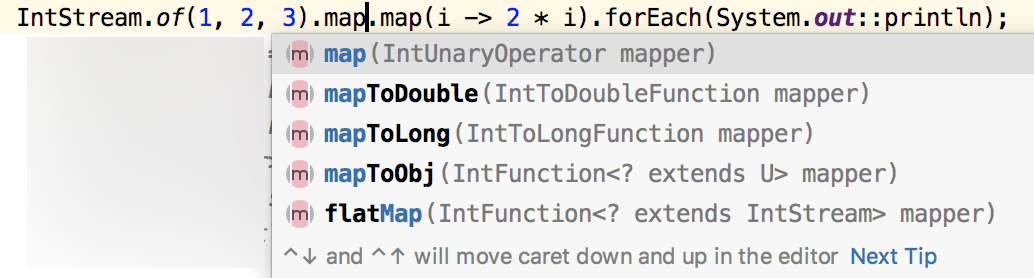# WebFlux 前置知识（三）

[TOC]

Stream 流式 API 也是 JDK8 开始引入的 API，用过的小伙伴都说爽，没用过的小伙伴则感到一脸懵逼。现在 JDK 已经到 16 了，可能很多人还不熟悉 Stream 流操作，这次趁着讲 WebFlux，松哥也来和大家一起回顾一下 Stream 流操作。

## 1.Stream 流简介

Java8 中的 Stream 不存储数据，它通过函数式编程模式来对集合进行链状流式操作。

## 2.基本玩法

int[] arr = {1, 2, 3, 4, 5, 6};


double asDouble = IntStream.of(arr).average().getAsDouble();


int sum = IntStream.of(arr).sum();


double[] arr = {1, 2, 3, 4, 5, 6};
double sum = DoubleStream.of(arr).map(i -> Math.pow(i, 2)).sum();


• 中间操作
• 终止操作

## 3.Stream 的创建

### 集合

List 集合或者 Set 集合都可以直接搞一个流出来，方式如下：

List<String> list = new ArrayList<>();
Stream<String> s1 = list.stream();
Set<String> set = new HashSet<>();
Stream<String> s2 = set.stream();


### 数组

IntStream stream = Arrays.stream(new int[]{11, 22, 33, 44, 55, 66});


### 数字 Stream

IntStream s1 = IntStream.of(1, 2, 3);
DoubleStream s2 = DoubleStream.of(1, 2, 3);
LongStream s3 = LongStream.of(1L, 2L, 3L);


### 自己创建

Random random = new Random();
Supplier<Integer> supplier = () -> random.nextInt(100);
Stream<Integer> stream = Stream.generate(supplier).limit(5);


## 4.Stream 的中间操作

• map 或者 filter 会从输入流中获取每一个元素，并且在输出流中得到一个结果，这些操作没有内部状态，称为无状态操作。
• reduce、sum、max 这些操作都需要内部状态来累计计算结果，所以称为有状态操作。

• map/mapToXxx
• flatMap/flatMapToXxx
• filter
• peek

• distinct
• sorted
• limit/skip

map

Stream.map() 是 Stream 中最常用的一个转换方法，可以把一个 Stream 对象转为另外一个 Stream 对象。map 方法所接收的参数就是一个 Function 对象，松哥在前面文章中和大家介绍过 Function 对象了，就是有输入有输出（参见WebFlux 前置知识（一）），了解了 map 的参数，那么 map 的功能就很明白了，就是对数据进行二次加工。

String[] arr = {"1", "2", "3"};
Stream<String> s1 = Arrays.stream(arr);
Stream<Integer> s2 = s1.map(i -> Integer.valueOf(i));


IntStream.of(1, 2, 3).map(i -> 2 * i).forEach(System.out::println);


JDK 中也提供了一些现成的格式转换，如下图：String[] arr = {"1", "2", "3"};
Stream<String> s1 = Arrays.stream(arr);
s1.mapToLong(i -> Long.parseLong(i)).forEach(System.out::println);


flatMap

flatMap 可以把 Stream 中的每个元素都映射为一个 Stream，然后再把这多个 Stream 合并为一个 Stream。

Stream<Integer[]> s = Stream.of(new Integer[]{1, 2, 3}, new Integer[]{4, 5, 6}, new Integer[]{7, 8, 9});
s.forEach(System.out::println);


Stream<Integer> s = Stream.of(new Integer[]{1, 2, 3}, new Integer[]{4, 5, 6}, new Integer[]{7, 8, 9}).flatMap( i -> Arrays.stream(i));
s.forEach(System.out::println);


filter

filter 操作会对一个 Stream 中的所有元素一一进行判断，不满足条件的就被过滤掉了，剩下的满足条件的元素就构成了一个新的 Stream。

IntStream.of(2, 3, 4, 5, 6, 7).filter(i -> i > 3).forEach(System.out::println);


filter 方法接收的参数是 Predicate 接口函数，关于 Predicate 接口函数，大家可以参考WebFlux 前置知识（一））一文。

peek

peek 的入参是 Consumer，没有返回值，因此当我们要对元素内部进行处理时，使用 peek 是比较合适的，这个时候可以不用 map（map 的入参是 Function，它是有返回值的）。peek 方法本身会继续返回流，可以对数据继续进行处理。

IntStream.of(2, 3, 4, 5, 6, 7).filter(i -> i > 3).peek(String::valueOf).forEach(i-> System.out.println(i));


peek 方法的感觉就像数据中途被消费了一次。

distinct

IntStream.of(2, 3, 4, 3, 7, 6, 2, 5, 6, 7).distinct().forEach(System.out::println);


sorted

sorted 是排序，因为也需要知道其他元素的值，然后才能去重，所以这个也是有状态操作，如下：

IntStream.of(2, 3, 4, 3, 7, 6, 2, 5, 6, 7).distinct().sorted().forEach(System.out::println);


limit/skip

limit 和 skip 配合操作有点像数据库中的分页，skip 表示跳过 n 个元素，limit 表示取出 n 个元素。例如下面这个例子：

Arrays.asList('A', 'B', 'C', 'D', 'E', 'F').stream().skip(2).limit(3).forEach(System.out::println);


## 5.Stream 终止操作

• 短路操作：不用处理全部元素就可以返回结果。
• 非短路操作：必须处理所有元素才能得到最终结果。

• forEach/forEachOrdered
• collect/toArray
• reduce
• min/max/count

• findFirst/findAny
• allMatch/anyMatch/noneMatch

forEach/forEachOrdered

forEach 和 forEachOrdered 都是接收一个 Consumer 类型的参数，完成对参数的消费，不同的是，在并行流中，forEachOrdered 会保证执行顺序。

int[] arr = {1, 2, 3, 4, 5, 6, 7, 8, 9};
Arrays.stream(arr).parallel().forEach(System.out::println);
Arrays.stream(arr).parallel().forEachOrdered(System.out::println);


collect/toArray

List<Integer> list = Stream.of(1, 2, 3, 4).filter(p -> p > 2).collect(Collectors.toList());
System.out.println(list);


reduce

reduce 是 Stream 的一个聚合方法，它可以把一个 Stream 的所有元素按照聚合函数聚合成一个结果。reduce 方法传入的对象是BinaryOperator 接口，它定义了一个apply 方法，负责把上次累加的结果和本次的元素进行运算，并返回累加的结果。

Optional<Integer> optional = Stream.of(1, 2, 3, 4).reduce((i, j) -> i + j);
System.out.println(optional.orElse(-1));


reduce 的参数是 BinaryOperator，这个接收两个参数，第一个参数是之前计算的结果，第二个参数是本次参与计算的元素，两者累加求和。

Optional<String> s = Stream.of("wwwjavaboyorg".split("")).reduce((i, j) -> i + "." + j);
System.out.println(s.orElse(""));


w.w.w.j.a.v.a.b.o.y.o.r.g


min/max/count

Stream<Integer> s = Stream.of(1, 2, 3, 4);
long count = s.count();
System.out.println("count = " + count);


Stream<Integer> s = Stream.of(1, 2, 3, 4);
Optional<Integer> min = s.min(Comparator.comparingInt(i -> i));
System.out.println("min.get() = " + min.get());


findFirst/findAny

for (int i = 0; i < 10; i++) {
Optional<Integer> first = Stream.of(1, 2, 3, 4).parallel().findFirst();
System.out.println("first.get() = " + first.get());
}
System.out.println("=============");
for (int i = 0; i < 10; i++) {
Optional<Integer> first = Stream.of(1, 2, 3, 4).parallel().findAny();
System.out.println("first.get() = " + first.get());
}


allMatch/anyMatch/noneMatch

allMatch、anyMatch、noneMatch 用来判断所有元素、任意元素或者没有元素满足给定的条件。这三个方法的参数都是一个 Predicate 接口函数。

boolean b = Stream.of(1, 2, 3, 4).allMatch(i -> i > 5);
System.out.println("b = " + b);


## 6.并行流

new Random().ints().limit(50).parallel().forEach(i->{
});


## 7.收集器

• 提取出用户对象中的 age 属性组成新的集合并返回。
• 提取出用户对象中的 gender 属性组成新的集合并返回（这里是一个 Set 集合，所以会自动去重）。
List<Integer> ages = users.stream().map(User::getAge).collect(Collectors.toList());
System.out.println("ages = " + ages);
Set<String> genders = users.stream().map(User::getGender).collect(Collectors.toSet());
System.out.println("genders = " + genders);


Collectors.toList() 最终返回的是 ArrayList，Collectors.toSet() 最终返回的是 HashSet。

List<Integer> ages = users.stream().map(User::getAge).collect(Collectors.toList());
System.out.println("ages = " + ages);
TreeSet<String> genders = users.stream().map(User::getGender).collect(Collectors.toCollection(TreeSet::new));
System.out.println("genders = " + genders);


IntSummaryStatistics ageStatistics = users.stream().collect(Collectors.summarizingInt(User::getAge));
System.out.println("ageStatistics = " + ageStatistics);


ageStatistics = IntSummaryStatistics{count=20, sum=1222, min=9, average=61.100000, max=96}


Map<Boolean, List<User>> map = users.stream().collect(Collectors.partitioningBy(u -> u.getGender().equals("男")));
System.out.println("map = " + map);


Map<String, List<User>> map2 = users.stream().collect(Collectors.groupingBy(User::getGender));
System.out.println("map2 = " + map2);


Map<String, Long> map2 = users.stream().collect(Collectors.groupingBy(User::getGender,Collectors.counting()));
System.out.println("map2 = " + map2);• 微信咨询